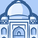# New to Qlik Sense

If you’re new to Qlik Sense, start with this Discussion Board and get up-to-speed quickly.

Announcements
Customer & Partners, DEC. 9, 11 AM ET: Qlik Product & Strategy Roadmap Session: Data Analytics REGISTER NOW
cancel
Showing results for
Did you mean:Contributor III

## Dynamically calculate variable with measure in table where dimension is dynamically defined as date difference

Hi Qlik Sense experts 🙂

I have one date column in data and one variable where user can define the second date.

Source table is like

ID, AR_Due_Date, AR_Amount

1, 2017-12-31, 10

2, 2017-11-22, 20

And variable is user defined in dashboard \$(vAsOfDateAR)

Those 2 columns use to calculate dimension date difference <1 to calculate date ranges

=if((Date(\$(=num(Date#(vAsOfDateAR,'DD/MM/YYYY'))))-[AR_Due_Date]) <1, 'not overdue',

if((Date(\$(=num(Date#(vAsOfDateAR,'DD/MM/YYYY'))))-[AR_Due_Date]) <31,'1 - 30',

if((Date(\$(=num(Date#(vAsOfDateAR,'DD/MM/YYYY'))))-[AR_Due_Date]) <91,'31 - 90',

....

This works correctly in table as well as graph. I calculate table with sum(Amount) as measure.

But the task is following. The user would like to add addtional parameters (defined in dashboard as variables (eg vAR1-vAR6) where

each variable represents one segment defined in previous dimension.

The final result should be table where Measure (AR_Amount_in_CZK) is multiplied with those variables depending to which segment it belongs.

This logic doesn't work.

=if((Date(\$(=num(Date#(vAsOfDateAR,'DD/MM/YYYY'))))-[AR_Due_Date]) <1,\$(vAR1)*sum(AR_Amount_in_CZK),

if((Date(\$(=num(Date#(vAsOfDateAR,'DD/MM/YYYY'))))-[AR_Due_Date]) <31,\$(vAR2)*sum(AR_Amount_in_CZK),

if((Date(\$(=num(Date#(vAsOfDateAR,'DD/MM/YYYY'))))-[AR_Due_Date]) <91,\$(vAR3)*sum(AR_Amount_in_CZK)))

I attach printscreen where it is more visible

Problem is I am unable to match correct variable to correct segment to calculate final result.